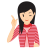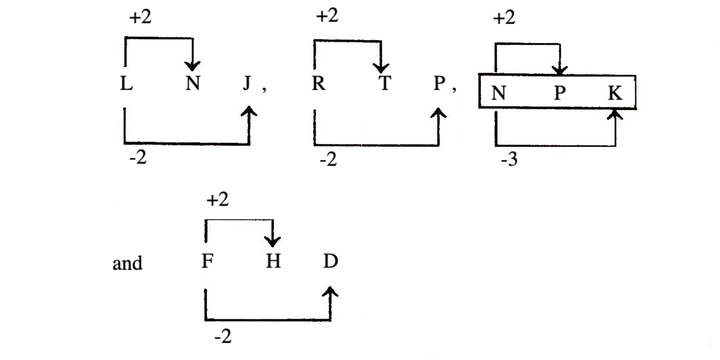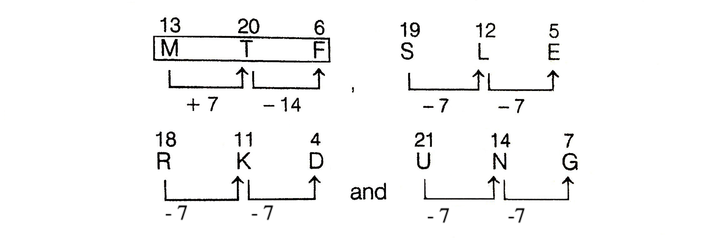# Classification

'Classification' means to group the given items on the basis of certain common quality that they possess.Few Important things to Remember

In this section, the questions consist of four/five elements. All the elements except one, follow a certain pattern/rule or have some common quality and thus form a group. The candidate has to find the element that does not belong to the group.

Classification may be broadly divided into the following types:

1. Word Classification
2. Number Classification
3. Letter Classification

## Word Classification

In word classification, the candidate is given a group of words in which all except one are related to one another in a particular manner thus form a group. The candidate has to identify the odd word that does not belong to the group.

Example 1: Choose the odd one.

(a) Square                    (b) Circle

(c) Parallelogram          (d) Rectangle

Solution:  (b) Except circle, all other given shapes are made up of straight lines.

Example 2: Choose the odd one.

(a) Eyes     (b) Noes     (c) Ears      (d) Lungs

Solution:  (d) Except lungs, all other given shapes are external body parts, but lungs are internal parts of body.

## Number Classification

In number classification, there is a similarity among the given numbers or group of numbers. The candidate has to identify the odd number or group of numbers which does not belong to the group.

The number classification is generally based on the following similarities:

• Square and square root of a number

• Cube and cube root of a number

• Even and odd number

• Prime number

• Divisibility test of a number

• Sum of digits of a number

Example 1: Choose the odd one.

(a) 27      (b) 35     (c) 18     (d) 9

Solution:  (b) Except 35, all others are multiples of 9, but 35 is the multiple of 5 and 7.

Example 2: Choose the odd one.

(a) 16 - 18   (b) 32 - 84  (c) 12 - 52  (d) 49 - 77

Solution:  (c)   2 x 8 = 16
8 x 4 = 32
7 x 7 = 49
But,                 5 x 2 = 10
Thus, 12 - 52 is an odd pair.

## Letter Classification

In letter classification, there is a similarity or pattern among the letters in the group and the candidate has to identify the pattern and select the one which does not follow the common pattern in the group.

The relationship among the letters is generally based on the following similarities:

• Position of letters

• Vowel and consonant

• Reverse order of letters

• Addition or multiplication of positional value

Example 1: Choose the odd one.

(a) H                   (b) Q

(c) T                    (d) Z

Solution:  (b) All other letters except Q, occupy the even-numbered positions in the English alphabets.

As,            H -----> 8 (even),     Q -----> 17 (odd)
T -----> 20 (even),    Z -----> 26 (odd)

Example 2: Choose the odd one.

(a) LNJ                    (b) RTP

(c) NPK                   (d) FHD

Solution:  (c)Clearly, letters group NPK follows different pattern from others.

Hence, NPK does not belong to the group.

MIND IT !

Besides word, letter and number classification, there also exits mixed classification in which combination of letters and numbers are given

Example 3: Choose the odd one.

(a) OE3     (b) XD6     (c) JB5      (d) VK2      (e) PH3

Solution:  (e) Here, putting the positional value of letters according to English alphabetical order as :

A = 1, B = 2, C = 3, ......, Z = 26
and solving the alternatives, we have

OE3 => OE = O ÷ E = 15 ÷ 5 = 3;
XD6 => XD = X ÷ D = 24 ÷ 4 = 6;
JB5 => JB = J ÷ B = 10 ÷ 2 = 5;
VK2 => VK = V ÷ K = 22 ÷ 11 = 2;
PH3 => PH = P ÷ H = 16 ÷ 8 = 2 ≠ 3

In PH3, the numerical value should be 2 instead of 3 because 16 ÷ 8 = 2.

Hence, PH3 does not belong to the group.

## Types of questions

There are several types of questions that can be asked from classification. Some of the types are discussed below:

Type #1: Choosing the Odd Word/Letter/Letter's Group/Number

In this type of problems, four/five words/letters/letters' groups/numbers are given having some common features except the odd one.
You are required to find the 'odd one out'.

Example 1: Choose the odd one.

(a) Strong                 (b) Hesitant

(c) Daring                 (d) Brave

Solution:  (b) Hesitant is different as all others are synonyms of each other.

Example 2: Choose the odd one.

(a) Marigold      (b) Tulip      (c) Lotus      (d) Rose

Solution:  (b) Except Tulip, all others are flowers whereas Tulip is a kind of plant.

Example 3: Choose the odd one.

(a) MTF                     (b) SLE

(c) RKD                     (d) UNG

Solution:  (a) Here, the letters' groups follow the below patternExcept MTF, all others follow similar pattern. So, letters' group MTF is different from others.

Example 4: Choose the odd one.

(a) HGFED                     (b) PONML

(c) NLKJI                         (d) TSRQP

Solution:  (c) Except option (c), in all the other groups the consecutive letters are given in reverse order But in option (c), letter M is missing.

Example 5: Choose the odd one.

(a) 144    (b) 169    (c) 256    (d) 288    (e) 324

Solution:  (d) Except 288 all other numbers are square of natural numbers.

As, 122 = 144, 132 = 169

162 = 256, 182 = 324

Example 6: Choose the odd one.

(a) 512    (b) 625    (c) 1296    (d) 2401

Solution:  (a) Here, 512 is the cube of 8, whereas other numbers are square of 25, 36 and 49, respectively. So 512 is different from others.

Type #2: Choosing the Odd Pair of Words/Numbers

In this type of questions, different pairs of words/numbers having some common features/properties like names, places, uses, situations, origin, operations etc. are given, except one pair.
You have to identify the odd pair.

Example 1: Choose the odd pair.

(a) Gold : Ornaments

(b) Cloth : Garments

(c) Wood : Furniture

(d) Leather : Footwear

(e) Earthen pots: Clay

Solution:  (e) Except pair (e), in all other pairs, the first is the raw material used to make the second.

Example 2: Choose the odd pair.

(b) Light - Heavy

(c) Tiny - Small

(d) Big - Large

Solution:  (b), Light is an antonym of heavy. But in other pairs, words are synonyms of each other.

Example 2: Choose the odd pair.

(a) (21 : 24)

(b) (28 : 32)

(c) (14 : 16)

(d) (70 : 80)

(e) (54 : 62)

Solution:  (e) Here ,    21/24 = 7/8 ; 28/32 = 7/8;
14/16 = 7/8; 70/80 = 7/8
and                              54/62 = 27/31

Clearly, 54 : 62 is different from others.

LearnFrenzy provides you lots of fully solved Classification Questions and Answers with explanation.